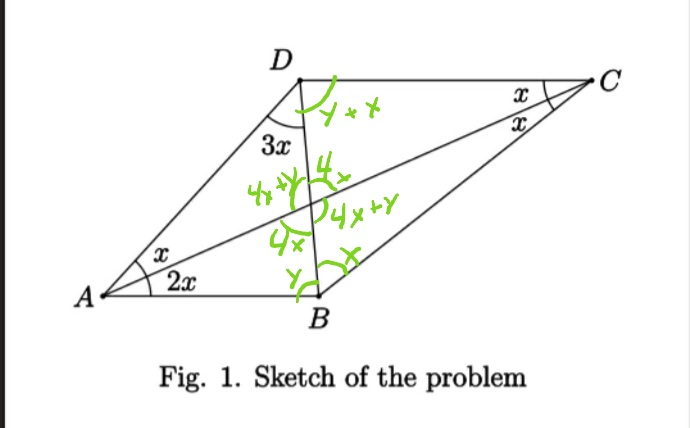# 🔒 How do you find the angle inside a kite?

I found this problem in my geometry textbook and I’ve been staring at the picture for a while and I am out of ideas.According to the official answers sheet the answer is 15 degrees given in sexagesimal units.

The rules for this is that you may not use trigonometry or triangle similarity. So in essence is a puzzle. Well, i am not good with constructions. Does any of you guys can help me?

You can use isosceles triangles, circles, equilateral triangles, congruence and all of those sorts. Well, not the secant tangent formula, but I doubt that it will be needed.

Since this problem is in a section where it only introduces concepts like midpoint of a triangle, congruence cases, and basic theorems like incenter, circumcenter, barycenter and orthocenter. Maybe using these simple tools it can be solved.

Therefore, Is there a math wiz who can someone help me?. Please say it with some logical steps without skipping something so I don’t get lost.

What’s the question asking? I only see that the answer is “15 degrees given in sexagesimal units.”

I thought it would be obvious but it is asking find the value of x.

I don’t know the answer but the value of all angles inside the kits need to ad up to 360 since it is complete.

You can infer that 2x it 2 times greater than x. And therefore 3 x is 3 times greater than x.

1 Like

There’s two unknown angles in there. Assign them variable names, then you can form three simultaneous equations in three variables using the basic properties of a triangle and square.

1 Like

Oh, God. I’m in a rabbit hole, learning about the Babylonians. Time to put down the phone and get some work done.

am now depressed becoz cannot maf

2 Likes

I’m on mobile getting ready work, but I was able to work out the angles using properties of triangle:

• The sum of all angles of a triangle equal 180
• Opposite angles have the same valueReminding that the sum of the angles of a quadrilateral is 360, I think you can take from there.

2 Likes

edit: What I wrote was nonsense. As shown by the pictures Ender drew: How do you find the angle inside a kite? - #71 by Ender. It probably only works for x = 15 degree. Hopefully someone educated can explain how to reach the solution. Considering the trickiness of the problem, it’s probably contest math question.

But you haven’t got “there”. It should be `2x + y` instead of `4x + y`, where `y` is the angle ABD. And angle DBC should be `3x`.

Anyways, it doesn’t tell us much. It just gives us the equation `y + 6x = 180`. Which we could already tell from triangle ABD.

I suspect the solution is that line equation `y + 6x = 180` subject to certain constraints like, `y` and `x` should be positive, and the interior angles should be between 0 and 180 degrees.

Because if we follow the suggested solution, `x` is `15 degrees`, and `y` would be `90 degrees`. But other pairs like `x = 10 deg` and `y = 120 deg`, etc. seem to work just fine.

Perhaps the question is missing certain information. But then again, I have no idea what “degrees given in sexagesimal units” means.

1 Like

Fuck the angles will the bitch fly is what I want to know…

4 Likes

Well it looks like angle A has to be 3x, and so is angle D, and so it follows that A and D is the same value. angle C has to be 2x and therefore assuming angle C is 30 degrees (which is what it would be if the answer is 15), then angle A and D must both be 45 degrees. But then that means the remaining angle of triangle ABD must be 90 degrees but the text does not indicate that it is (if you have a triangle with 2 45 degree angles then the last angle MUST be 90 degrees, otherwise it won’t work), but inferring from the diagram it looks like angle B must be an acute angle if you don’t include the other side of the triangle.

In the end you should probably plug this into wolfram alpha and see what it tells you, or use calculus.

1 Like

This is confusing. I thought that the way we normally express degrees as degrees, minutes, seconds already was expressed as sexagesimal units, as opposed to being expressed as, say, radians.

2 Likes

Or pieces of pie.

2 Likes

I knew that something was wrong, but I decided to post anyway.
You know, Cunningham’s LawAre AB and DC parallel?

As I’m on my computer its actually quicker for me to draw it up and measure it than to work it out.

Yeah, quicker maybe, but that doesn’t give you the right answer lol. You can’t assume the diagram is to scale.

For example, you’ve calculated four different values for x there (14.6°, 15.8°, 23.6°, and 23.7°).

4 Likes

I don’t think you can assume that.

They are the angles for that exact drawing (via Autocad), so my conclusions are, is x is not a constant or that can’t draw.

What if the drawing is not on scale?